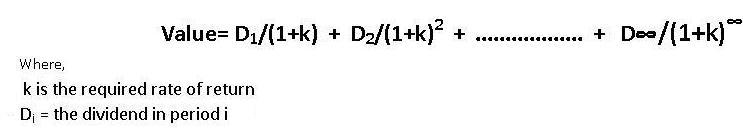# Finance Assignment Help With Approaches To Equity Valuation

## Approaches To Equity ValuationThere are two main approaches to equity valuation. The first is using discounted cash flow techniques and the second is using relative measures (e.g. price/earnings and price/book value ratios). The first method we look at is the dividend discount model which is when we consider the value of the cash flows (dividends) that are paid to the equity investor.

### Dividend discount model

This model values equities as the present value of future dividends to be paid to the investor.

###If it can be assumed that:

• The share is going to be held indefinitely
• The growth rate (g) of dividends is constant
• k is greater than g

Then the equation can be simplified to:

### Following are some of the topics in Stocks in which we provide help:

Approaches To Equity Valuation Assignment Help | Corporate Finance Homework Help | Finance Assignment Help | Finance Assignment Help | Finance Homework Help | Finance Online Help | Finance Problems Help | Finance Tutor | Help With Finance Homework | Online Tutoring